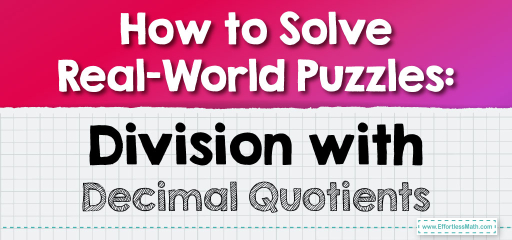# How to Solve Real-World Puzzles: Division with Decimal Quotients

In the real world, numbers don't always neatly divide into whole numbers. Often, we encounter situations where the result of a division is a decimal. Let's explore some word problems that involve division leading to decimal quotients.## Division Using Decimal Quotients: Word Problems

### Example 1:

John has $$5$$ apples, and he wants to share them equally among his $$3$$ friends. How many apples does each friend get?

Solution Process:

Divide the number of apples (5) by the number of friends (3).

Each friend gets $$1.67$$ apples, or $$1$$ apple and $$2/3$$ of another apple.

The Absolute Best Book for 5th Grade Students

### Example 2:

A chocolate bar costs $$4$$ dollars. If Lisa has $$7$$ dollars, how many chocolate bars can she buy?

Solution Process:

Divide the amount Lisa has (7) by the cost of one chocolate bar (4).

Lisa can buy $$1.75$$ chocolate bars, or $$1$$ chocolate bar and three-quarters of another one.

Word problems involving division with decimal quotients bring math to life. They help us understand how mathematical concepts apply to everyday situations. By practicing these problems, you’ll not only sharpen your division skills but also develop a keen sense of how math operates in the real world. So, the next time you’re faced with a real-world division problem, approach it with confidence and let your mathematical prowess shine!

### Practice Questions:

1. Mike ran $$8$$ kilometers in $$3$$ hours. How many kilometers did he run per hour?

2. A bottle contains $$11$$ liters of water. If $$5$$ people share it equally, how many liters does each person get?

3. A pizza has $$10$$ slices. If $$6$$ friends share it equally, how many slices does each friend get?

4. A book costs $$9$$ dollars. If you have $$15$$ dollars, how many books can you buy?

A Perfect Book for Grade 5 Math Word Problems!

1. $$2.67$$ kilometers per hour.

2. $$2.2$$ liters per person.

3. $$1.67$$ slices or $$1$$ slice and $$2/3$$ of another slice.

4. $$1.67$$ books or $$1$$ book and $$2/3$$ of another book.

The Best Math Books for Elementary Students

### What people say about "How to Solve Real-World Puzzles: Division with Decimal Quotients - Effortless Math: We Help Students Learn to LOVE Mathematics"?

No one replied yet.

X
51% OFF

Limited time only!

Save Over 51%

SAVE $15 It was$29.99 now it is \$14.99